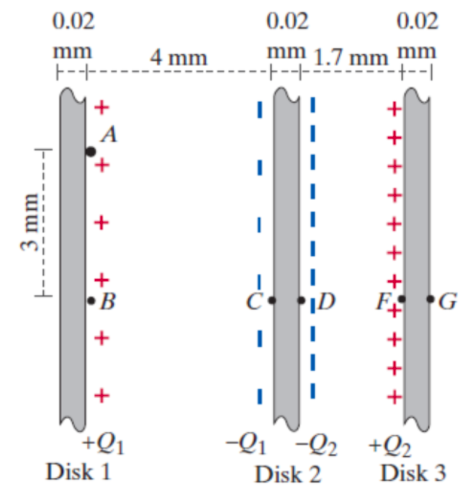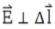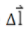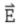# Problem: Three charged metal disks are arranged as shown (cutaway view). The disks are held apart by insulating supports not shown in the diagram. Each disk has an area of 2.3 m2 (this is the area of one flat surface of the disk). Use the value 8.85x10-12 C2/(N·m2) for ε0.The charge Q1 = 5x10-8 coulombs, and the charge Q2 = 2x10-7 coulombs.1) What is the magnitude of the electric field in the region between disks 1 and 2? |E12| =________________V/m2) What is the direction of the electric field between disks 1 and 2?a. +xb. -xc. +yd. -ye. +zf. -z3) Which of the following statements are true? Choose all that apply.a. VB - VA =0b Along a path from A to B c. VB - VA = = - ((Q1/2.3)/ε0)*(0.003) volts4) To calculate VC - VB, which path should you choose?a. A path starting at B and ending at Cb. A path starting at C and ending at Bc. It doesn't matter whether the path starts or ends at B5) The sign of VC - VB should be:a. Zero - no sign.b. Negative, because  is in the same direction as .c. Positive, because  is opposite to the direction of .6) What is the potential difference VC - VB? VC - VB = ______________________________volts7) What is the potential difference VD - VC? VD - VC =____________________ volts8) What is the potential difference VF - VD? VF - VD =____________________ volts9) What is the potential difference VG - VF?VG - VF = ___________________ volts10) What is the potential difference VG - VA?VG - VA = _________________voltsThe charged disks have tiny holes which allow a particle to pass through them. An electron which is traveling at a fast speed approaches the plates from the left side. It travels along a path from A to G. Since no external work is done on the system of plates + electron, Δ K + ΔU = Wext = 0 Consider the following states: Initial, electron at location A. Final, electron at location G.11) What is the change in potential energy of the system? ΔU =_________________________________ joules12) What is the change in kinetic energy of the electron? ΔK =_________________________________ joules

###### FREE Expert Solution

The separated plates form a parallel plate capacitor.

The capacitance of a parallel plate capacitor:

$\overline{){\mathbf{C}}{\mathbf{=}}\frac{{\mathbf{\epsilon }}_{\mathbf{0}}\mathbf{A}}{\mathbf{d}}}$ k is the dielectric constant of the material between the plates, A is the area, and d is plate separation.

Electric field:

$\overline{){\mathbf{E}}{\mathbf{=}}\frac{\mathbf{V}}{\mathbf{d}}}$

81% (485 ratings)###### Problem Details

Three charged metal disks are arranged as shown (cutaway view). The disks are held apart by insulating supports not shown in the diagram. Each disk has an area of 2.3 m2 (this is the area of one flat surface of the disk). Use the value 8.85x10-12 C2/(N·m2) for ε0.The charge Q1 = 5x10-8 coulombs, and the charge Q2 = 2x10-7 coulombs.1) What is the magnitude of the electric field in the region between disks 1 and 2?

|E12| =________________V/m

2) What is the direction of the electric field between disks 1 and 2?
a. +x
b. -x
c. +y
d. -y
e. +z
f. -z

3) Which of the following statements are true? Choose all that apply.
a. VB - VA =0
b Along a path from A to Bc. VB - VA = = - ((Q1/2.3)/ε0)*(0.003) volts

4) To calculate VC - VB, which path should you choose?
a. A path starting at B and ending at C
b. A path starting at C and ending at B
c. It doesn't matter whether the path starts or ends at B

5) The sign of VC - VB should be:
a. Zero - no sign.
b. Negative, becauseis in the same direction as.
c. Positive, becauseis opposite to the direction of.

6) What is the potential difference VC - VB? VC - VB = ______________________________volts

7) What is the potential difference VD - VC? VD - V=____________________ volts

8) What is the potential difference VF - VD? VF - VD =____________________ volts

9) What is the potential difference VG - VF?
VG - VF = ___________________ volts

10) What is the potential difference VG - VA?
VG - VA = _________________volts

The charged disks have tiny holes which allow a particle to pass through them. An electron which is traveling at a fast speed approaches the plates from the left side. It travels along a path from A to G. Since no external work is done on the system of plates + electron, Δ K + ΔU = Wext = 0 Consider the following states: Initial, electron at location A. Final, electron at location G.

11) What is the change in potential energy of the system?

ΔU =_________________________________ joules

12) What is the change in kinetic energy of the electron?

ΔK =_________________________________ joules